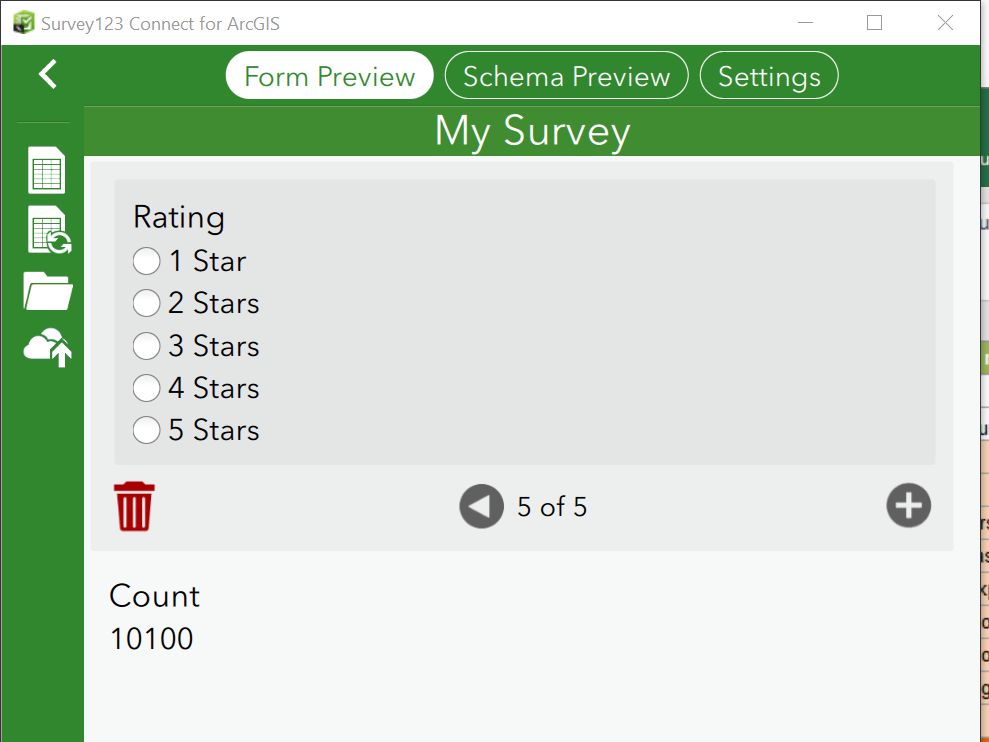# Count unique instances of a value within a repeat using SUM

315
1
11-08-2018 03:35 PM
Highlighted
New Contributor III

I am wanting to count the unique instances of a value within a repeat. Others have had the same issues resolved by explicitly casting the values as integers but this isn't resolving my issue.

I have a calculate field based on a conditional IF statement such that if the condition is met a value is set. Outside of the repeat I have a sum of the conditional field. But instead of summing the integers, the output is a concatenation of text despite trying to cast values as integers and binding as an esriFieldTypeInteger.

if(condition),1,0)

sum(FIELD)

Attached is this example.

e.g.Instead of the value 2, I have 10100

 type name label calculation bind::esri:fieldType begin repeat Ratings select_one rating Rating Rating calculate Count_1_Star if(\${Rating}=1,int(1),int(0)) esriFieldTypeInteger integer note Note \${Count_1_Star} end repeat integer Integer_Count Count sum(\${Count_1_Star}) esriFieldTypeInteger1 Solution

Accepted Solutions
Highlighted
New Contributor III

I changed the 'type' of field to an 'integer' from 'calculate' and it works now. I will note that the 'esriFieldTypeInteger' designation has no impact, rather, it is the 'type' field that is significant as explained in Esri custom columns—Survey123 for ArcGIS | ArcGIS  .

Alternately, I can keep the 'type' field as 'calculate' and specify the data type in the 'bind::type' field.

 type name label calculation bind::type begin repeat Ratings select_one rating Rating Rating calculate Count_1_Star 1_star if(\${Rating}=1,int(1),int(0)) int end repeat integer Integer_Count Count sum(\${Count_1_Star}) int